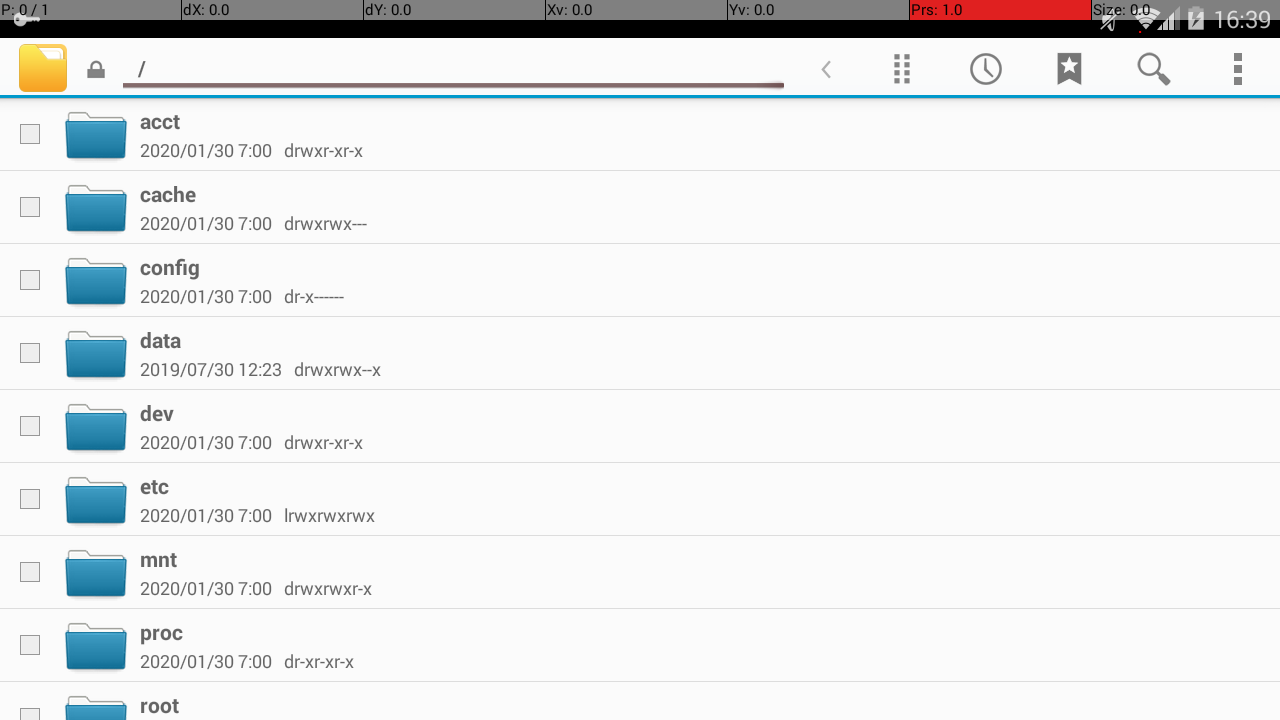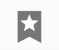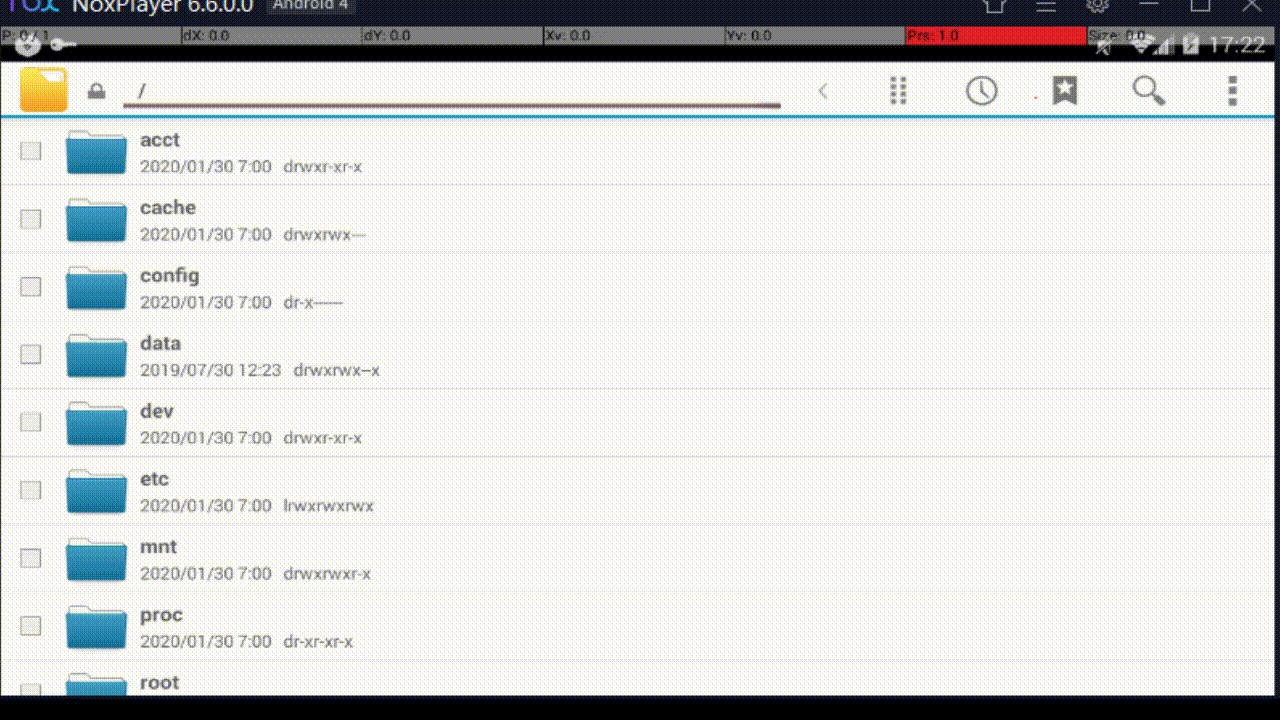# The basics of running NoxPlayer in Python

## background

Since the distribution and support of UWSC is over, I rewrote the macro that I wrote in UWSC before in Python. So, of the rewritten contents, I decided to summarize the important contents for running NoxPlayer with macros.

The contents include how to send ADB commands required for Nox operation, bidirectional operation between program and Nox, and fuzzy image recognition method. I put the source code, but it may be wrong because I wrote it while modifying it

## environment

NoxPlayer 6.6.0.0 Python 3.6 opencv-python 4.1.2.30

## Send and operate ADB commands

When automating Android operation with NoxPlayer, it is troublesome to operate the mouse one by one Therefore, it is necessary to operate in the background, but for that, it is necessary to use the ADB command. → Nox comes with nox_adb.exe as standard, so use this

First, in Python, the UWSC DOSCMD function can be written as:

#### `code`

``````
from subprocess import run, PIPE
def doscmd(directory, command):
completed_process = run(command, stdout=PIPE, shell=True, cwd=directory, universal_newlines=True, timeout=10)
return completed_process.stdout
``````

When sending ADB commands using this doscmd, for example, in the case of tap operation

#### `code`

``````
_dir = "D:/Program Files/Nox/bin"
return doscmd(_dir, cmd)

def tap(x, y):
_cmd = "nox_adb shell input touchscreen tap " + str(x) + " " + str(y)
``````

Can be described concisely Other ADB commands will come out if you check them (although you can put them later)

## See the behavior with logcat

When automating, it will be better if you can dynamically see the behavior of the application and Android with logcat, but at that time

#### `code`

``````
def show_log():
_cmd = "nox_adb logcat -d"
return _pipe
``````

Will output logcat For example, to open the Nox standard file manager with the ADB command and confirm that it has been opened

#### `code`

``````
def start_app():
_cmd = "nox_adb shell am start -n com.cyanogenmod.filemanager/.activities.NavigationActivity"
print(_pipe)

start_app()
``````

#### `Terminal`

``````
Starting: Intent { cmp=com.cyanogenmod.filemanager/.activities.NavigationActivity }
``````

But using logcat

#### `code`

``````
def clear_log():
_cmd = "nox_adb logcat -c"

def get_log():
_cmd = "nox_adb logcat -v raw -d -s ActivityManager:I | find \"com.cyanogenmod.filemanager/.activities.NavigationActivity\""
print(_pipe)

clear_log()
start_app()
get_log()
``````

#### `Terminal`

``````
START u0 {act=android.intent.action.MAIN cat=[android.intent.category.LAUNCHER] flg=0x10200000 cmp=com.cyanogenmod.filemanager/.activities.NavigationActivity bnds=[334,174][538,301](has extras)} from pid 681
Start proc com.cyanogenmod.filemanager for activity com.cyanogenmod.filemanager/.activities.NavigationActivity: pid=14454 uid=10018 gids={50018, 1028, 1015, 1023}
START u0 {act=android.intent.action.MAIN cat=[android.intent.category.LAUNCHER] flg=0x10200000 cmp=com.cyanogenmod.filemanager/.activities.NavigationActivity bnds=[334,174][538,301](has extras)} from pid 681
Start proc com.cyanogenmod.filemanager for activity com.cyanogenmod.filemanager/.activities.NavigationActivity: pid=14562 uid=10018 gids={50018, 1028, 1015, 1023}
START u0 {flg=0x10000000 cmp=com.cyanogenmod.filemanager/.activities.NavigationActivity} from pid 14665
Start proc com.cyanogenmod.filemanager for activity com.cyanogenmod.filemanager/.activities.NavigationActivity: pid=14675 uid=10018 gids={50018, 1028, 1015, 1023}
START u0 {flg=0x10000000 cmp=com.cyanogenmod.filemanager/.activities.NavigationActivity} from pid 14771
START u0 {act=android.intent.action.MAIN cat=[android.intent.category.LAUNCHER] flg=0x10200000 cmp=com.cyanogenmod.filemanager/.activities.NavigationActivity bnds=[334,174][538,301](has extras)} from pid 681
Start proc com.cyanogenmod.filemanager for activity com.cyanogenmod.filemanager/.activities.NavigationActivity: pid=14792 uid=10018 gids={50018, 1028, 1015, 1023}
``````

You can see the behavior like this

When automating application operations, you can do various things by looking at the timing of Activity transition and GC operation (memory release). clear_log-> concrete operation-> get_log-> discrimination, you can judge the screen state without image recognition </ font>

## Tap the specified image on the screen

When automating an application, objects move dynamically in ~~ games, etc., so the objects must be recognized programmatically. Therefore, by making the object image-recognized, it can be processed programmatically. As a concrete method

1. Take a screenshot of the screen with the ADB command Based on the screenshot in 2.1, let OpenCV recognize the image (template) for the corresponding image.
2. Determine the operation based on image recognition

Was the simplest method

As an advantage - Works in the background even if the window is minimized </ font> -Image recognition works regardless of the window size, and it is easier to take coordinates.

### step 1

When taking a screenshot in the image with the ADB command and saving it inside the PC

#### `code`

``````
_DIR_ANDROID_CAPTURE = "/sdcard/_capture.png "
_NAME_INTERNAL_CAPTURE_FOLDER = "pics"

def capture_screen(dir_android, folder_name):
_cmd = "nox_adb shell screencap -p " + dir_android

_cmd = "nox_adb pull " + dir_android+ " " + folder_name

capture_screen(_DIR_ANDROID_CAPTURE, _NAME_INTERNAL_CAPTURE_FOLDER)
``````

Will generate D: /Program Files / Nox / bin / pics / _capture.png Also, when creating a template, if you create it based on this image, you can create it regardless of the window size of Nox.

### Step 2

Specifically, as shown below, a method of returning the center coordinates of the part that matches the template in the image can be considered. When there is a template of img / temp.png in the same directory as main.py

#### `code`

``````
import cv2
import numpy as np

_DIR_INTERNAL_CAPTURE = "D:/Program Files/Nox/bin/pics/_capture.png "
_DIR_TEMP = "img/temp.png "
_THRESHOLD = 0.9 #Degree of similarity

def get_center_position_from_tmp(dir_input, dir_tmp):

gray = cv2.cvtColor(_input, cv2.COLOR_RGB2GRAY)
temp = cv2.cvtColor(_temp, cv2.COLOR_RGB2GRAY)

_h, _w = _temp.shape

_match = cv2.matchTemplate(_input, _temp, cv2.TM_CCOEFF_NORMED)
_loc = np.where(_match >= _THRESHOLD)
try:
_x = _loc
_y = _loc
return _x + _w / 2, _y + _h / 2
except IndexError as e:
return -1, -1

get_center_position_from_tmp(_DIR_INTERNAL_CAPTURE , _DIR_TEMP)
``````

Can be written

### Step 3

If you want to tap the coordinates obtained in step 2, use the tap method and get_center_position_from_tmp method above.

#### `code`

``````
_DIR_INTERNAL_CAPTURE = "D:/Program Files/Nox/bin/pics/_capture.png "
_DIR_TEMP = "img/temp.png "

x,y = get_center_position_from_tmp(_DIR_INTERNAL_CAPTURE, _DIR_TEMP)
tap(x, y)
``````

Can be described as

As a concrete example, the following screentemplateIf you want to tap with, Take a screenshot, cut out the relevant part with GIMP, and put it in img / tmp.png </ font> After that, execute the capture_screen method → get_center_position_from_tmp method → tap method in the order of the program </ font> In other words, to summarize the past

#### `code`

``````
from subprocess import run, PIPE
import cv2
import numpy as np

_DIR_NOX = "D:/Program Files/Nox/bin"
_DIR_ANDROID_CAPTURE = "/sdcard/_capture.png "
_NAME_INTERNAL_CAPTURE_FOLDER = "pics"
_DIR_INTERNAL_CAPTURE = "D:/Program Files/Nox/bin/pics/_capture.png "
_DIR_TEMP = "img/temp.png " #I put the bookmark image here
_THRESHOLD = 0.9 #Degree of similarity

def main():
capture_screen(_DIR_ANDROID_CAPTURE, _NAME_INTERNAL_CAPTURE_FOLDER)
x, y = get_center_position_from_tmp(_DIR_INTERNAL_CAPTURE, _DIR_TEMP)
tap(x, y)

def capture_screen(dir_android, folder_name):
_cmd = "nox_adb shell screencap -p " + dir_android

_cmd = "nox_adb pull " + dir_android+ " " + folder_name

get_center_position_from_tmp(_DIR_INTERNAL_CAPTURE , _DIR_TEMP)

def get_center_position_from_tmp(dir_input, dir_tmp):

gray = cv2.cvtColor(_input, cv2.COLOR_RGB2GRAY)
temp = cv2.cvtColor(_temp, cv2.COLOR_RGB2GRAY)

_h, _w = _temp.shape

_match = cv2.matchTemplate(_input, _temp, cv2.TM_CCOEFF_NORMED)
_loc = np.where(_match >= _THRESHOLD)
try:
_x = _loc
_y = _loc
return _x + _w / 2, _y + _h / 2
except IndexError as e:
return -1, -1

def doscmd(directory, command):
completed_process = run(command, stdout=PIPE, shell=True, cwd=directory, universal_newlines=True, timeout=10)
return completed_process.stdout

return doscmd(_DIR_NOX, cmd)

def tap(x, y):
_cmd = "nox_adb shell input touchscreen tap " + str(x) + " " + str(y)

if __name__ == '__main__':
main()
``````

And the actual behavior isShould be

## bonus

### Prevent the log from disappearing

If the log disappears immediately after execution, you will not be able to see what you printed, so you need to make sure that the log remains. To do this, write a = input () at the end of the macro

#### `code`

``````
def main():
print("Start")

#Processing unit

print("Finish")
_a = input()

if __name__ == "__main__":
main()
``````

### The one used in the ADB command

#### `code`

``````
##Tap
def tap(x, y):
_cmd = "nox_adb shell input touchscreen tap " + str(x) + " " + str(y)

##Swipe
def swipe(x1, y1, x2, y2, seconds):
_millis = seconds * 1000
_cmd = "nox_adb shell input touchscreen swipe " + str(x1) + " " + str(y1) + " " \
+ str(x2) + " " + str(y2) + " " + str(_millis)

##Long tap
def long_tap(x, y, seconds):
swipe(x, y, x, y, seconds)

##App (activity) start
def start_app():
_cmd = "nox_adb shell am start -n com.hoge.fuga/.MyActivity"

##App stop
def start_app():
_cmd = "nox_adb shell am force-stop com.hoge.fuga"

def return_home():
_cmd = "nox_adb shell input keyevent KEYCODE_HOME"

##Change the date of the terminal (I wrote it because the information was not collected only by this method)
import datetime

def set_date(delta_days):
_ANDROID_DATE_FORMAT = "%Y%m%d.%H%M%S"
_day = datetime.datetime.today() - datetime.timedelta(days=delta_days)
_days_ago = _day.strftime(_ANDROID_DATE_FORMAT)
_cmd = "nox_adb shell date -s " + date_2days_ago
``````

### Make an exe file

Making an exe using Pyinstaller will make progress

Please refer to the following site (it is troublesome to write) ・ Executable file with PyInstaller --Qiita

See also below when using with Pycharm, the correct answer is to use External Tools of aerobiomat -Configuring Pycharm to run Pyinstaller

### Create a configuration file

The directory of Nox itself may change depending on the environment, but if it is converted to an exe file, it will not be possible to change the program. Therefore, it is necessary to create a config.ini file in advance. In this example, assuming that the exe file and config.ini are in the same directory

#### `main.py`

``````
import configparser

def main():
config_ini = configparser.ConfigParser()

print("start macro")

#Processing unit

print("finish macro")
_a = input()

if __name__ == "__main__":
main()
``````

#### `config.ini`

``````
[DEFAULT]
NoxDirectory = D:/Program Files/Nox/bin
``````

Can be written as